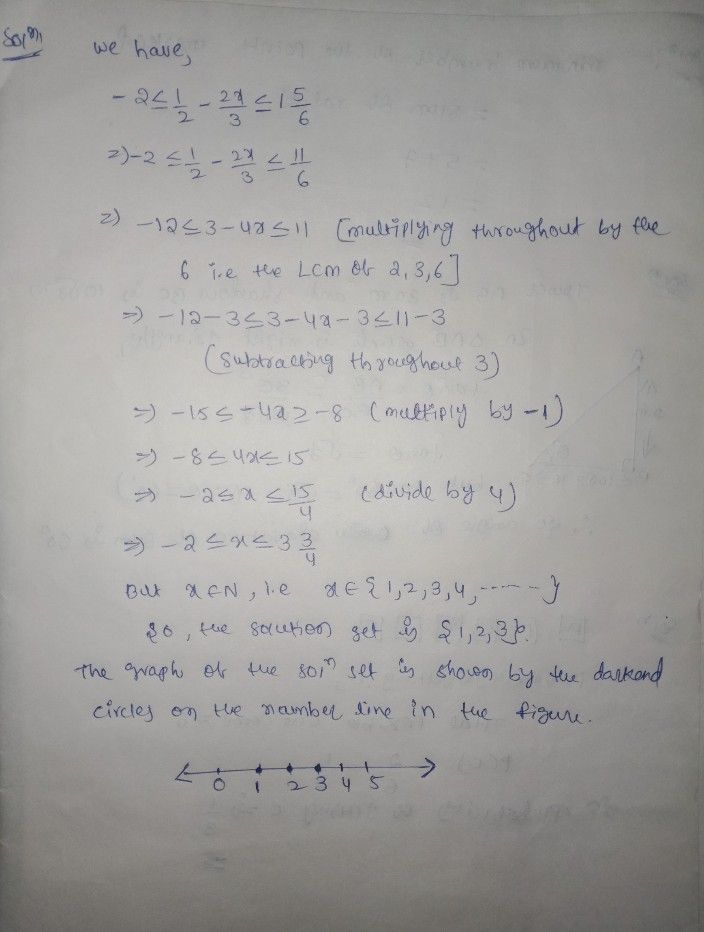Symbol
Problem$8$ Find the values of $x,$ which satisfy the inequation: $-2\leq \dfrac {1} {2}-\dfrac {2x} {3}\leq 1\dfrac {5} {6},x∈N$ Graph the solution on the number line.
7th-9th grade
English
SolutionQanda teacher - TeacherStudent
can I send you an exercise can you send me the Solutions of that exercise